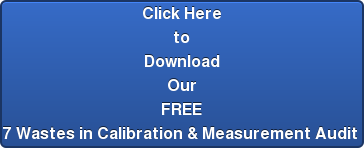••All circles are round, but not all round things are circles.

Conductivity and TDS (total dissolved solids) are often thought of a being interchangeable, but they are not. Conductivity is a measurement of the ability of a substance to conduct electricity. Its measurement is the reciprocal of Ohms, hence Mhos being a unit of conductivity (Ohms spelled backwards). So, if we are dealing with purely dissolved inorganic matter, think salts like sodium chloride, then the TDS can be obtained fairly accurately with a conductivity measurement.

Electrical conductivity of water is directly related to the concentration of dissolved ionized solids in the water. Ions from the dissolved solids in water create the ability for that water to conduct an electric current, which can be measured using a conventional conductivity meter or TDS meter. When correlated with laboratory TDS measurements, conductivity provides an approximate value for the TDS concentration, usually to within ten-percent accuracy. (Total dissolved solids, n.d.)

In the above passage notice the word correlated. It is used here because you cannot simply convert one unit to the other. We must measure the conductivity of the solution and then measure its TDS, we can then compare the two values and use conductivity to estimate the TDS of similar samples.

# How is TDS properly measured?

Gravimetric methods are the most accurate and involve evaporating the liquid solvent and measuring the mass of residues left. This method is generally the best, although it is time-consuming. If inorganic salts comprise the great majority of TDS, gravimetric methods are appropriate. (Total dissolved solids, n.d.)

As you could imagine carefully evaporating away the water from a sample to then weigh what is left over is a tedious process, but it is the only way to account for dissolved organic matter. Dissolved organic matter is not a particularly good conductor of electricity, however in the cases of things like stream water samples have been taken and analyzed over years to be able to estimate the TDS based on conductivity to approximate TDS to about 10%

# Conductivity

Conductivity is a much quicker measurement to perform. Two plates with a known distance between them have an electrical potential applied to them. The current can then be measured.

The SI unit of conductivity is siemens per meter. Conductivity measurements are used routinely in many industrial and environmental applications as a fast, inexpensive and reliable way of measuring the ionic content in a solution. For example, the measurement of product conductivity is a typical way to monitor and continuously trend the performance of water purification systems. (Conductivity, n.d.)Often encountered in industry is the traditional unit of μS/cm. The values in μS/cm are lower than those in μS/m by a factor of 100 (i.e., 1 μS/cm = 100 μS/m). Sometimes encountered is mho (reciprocal of ohm): 1 mho/m = 1 S/m. Historically, mhos antedate Siemens by many decades; good vacuum-tube testers, for instance, gave transconductance readings in micromhos. (Conductivity, n.d.)

# Conclusion

While conductivity and TDS are closely related as we’ve seen they are completely different measurements. Unfortunately, the manufacturers of conductivity meters for inorganic substances have been placing the label TDS on their devices and using conductivity units like ppm.

For a general rule of thumb, if your concern is measuring dissolved inorganic material like salts, a conductivity meter will give a reasonable approximation of the value, but if you are trying to measure TDS of an organic sample like pond water, the reading will not be accurate.

# Bibliography

Conductivity. (n.d.). Retrieved 7 11, 2017, from Wikipedia: The Free Encyclopedia: http://en.wikipedia.org/wiki/Conductivity_(electrolytic)

Image. (n.d.). Retrieved 7 11, 2017, from Wikipedia: The Free Encyclopedia: http://commons.wikimedia.org/wiki/File:Conductimetrie-schema.png

Kroenke, D., & Auer, D. (2009). Database Concepts. New Jersey: Prentice Hall.

Stair, R., & Reynolds, G. (2001). Principles of Information Systems. Boston: Course Technology.

Total dissolved solids. (n.d.). Retrieved 7 11, 2017, from Wikipedia: The Free Encyclopedia: http://en.wikipedia.org/wiki/Total_dissolved_solidsTopics: calibration

### Don't Miss Another Article!